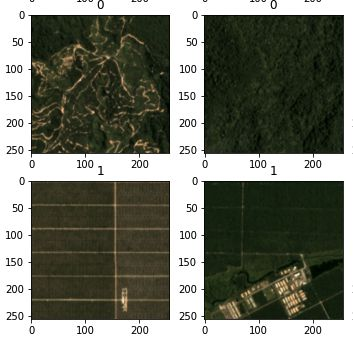# Feature Extraction via Transfer Learning and using predefined Model Architectures for Palm Plantation Identificationreport_website

This notebook is specifically structured to run/train/evaluate multiple models on the cluster. By setting the variables in the "setting" cell, it can be chosen whichmodels should be run and with wich general parameters. The logging of model information is handeld via "weights and bias". This structure has been chosen since quite a few GPU-intensive models are trained, the execution was therefore run on the university cluster instead of straiht from the notebook.
A detailed list of the models that have been run, their parameters and performance is summarized in the Report section.

# Content¶

2. Exploration
1.1 Visualization 1.2 Dataset Skewedness
4. untrained Architechtures (trained from scrach)
3.1 Training of Models
 3.1.1 LeNet5
3.1.2 ResNet16
3.1.2.1 ResNet16 "shallow end classifier"
3.1.2.2 ResNet16 "deep end classifier"
3.1.3 VGG18
3.1.4 Alexnet

3.2 Model Metrics
5. Pretrained Archtiechtures - Transfer Leraning
4.1 VGG
4.2 ResNet
6. Training of best model: ResNet16 trained from scratch
7. Report
6.1 Data - Quality and skewedness
6.2 untraines Models: Parameters and Performance
6.3 pretrained Models: Parameters and Performance
6.4 Final Model
6.5 Conclusion
In :
# imports
import wandb
import os
import pandas as pd
import numpy as np
import rasterio
from prettytable import PrettyTable

from sklearn.model_selection import train_test_split
import torch
from torch.utils.data import Dataset
from torchvision import datasets, transforms
from torchvision.transforms import ToTensor
import matplotlib.pyplot as plt
import random

import numpy as np
import pandas as pd
import matplotlib.pyplot as plt
from sklearn.model_selection import train_test_split

import IPython

import torch
import torch.nn as nn
import torch.nn.functional as F
import torch.optim as optim
import torchvision
from torchvision.transforms import transforms
import torchvision.datasets as datasets
from torchvision.transforms import ToTensor

from torch.optim import lr_scheduler
import time
import copy

In :
"""
SETTINGS
"""

# print and plot info
# if run inside a notebook, should be set to true
notebook = True

# choose to balance dataset
balance_dataset = True

# choose optimizer
optim = "sdg"

# define which of the models should be run
train_rf = False # train RF
train_lenet = False # train LeNet
train_resnet = False # train resnet
train_resnet_variation2 = False # train resnet
train_vgg = False # train vgg
train_alexnet = False # train alexnet
train_vgg_pretrained = False # retrain pretrained VGG
train_resnet_pretrained = False # retran pretrained resnet
train_and_save = True # train and save final model

# set data path
local_path = "/home/simon/CDE_UBS/deep_learning/EOT/assignment"
server_path = "/share/etud/e2008983/testing/assignment"
# set datapeth depending on if ru on server or lically
if os.path.exists(local_path):
project_path = local_path
if os.path.exists(server_path):
project_path = server_path


The information on the training data is stored in the csv file traindata.csv.

In :
train_df = pd.read_csv(os.path.join(project_path, "traindata.csv"))
if notebook:
print(train_df)

                  img_id  has_oilpalm
0     train/img_0000.jpg            0
1     train/img_0001.jpg            0
2     train/img_0002.jpg            0
3     train/img_0003.jpg            0
4     train/img_0004.jpg            0
...                  ...          ...
7672  train/img_7672.jpg            0
7673  train/img_7673.jpg            0
7674  train/img_7674.jpg            0
7675  train/img_7675.jpg            0
7676  train/img_7676.jpg            0

[7677 rows x 2 columns]


The img_id column indicates the relative path to the image and the has_oilpalm columns give the corresponding class index.

Let us now dowload the data and train a simple Random Forest algorithm on the flatten representation of the training images. As the data are big (~12GB if we donwload them in a float64 numpy array), we will use here only a subset of the data.

In :
#-- Training a RF model
if train_rf:

N = 500
#-- Getting the training dataset (X,y)
X = np.zeros((N,256*256*3), dtype=np.uint16)
y = np.zeros((N,), dtype=np.uint8)
train_rf_model = train_df.sample(n=N)
for n in range(N):
y[n] = train_rf_model.iloc[n]['has_oilpalm']

from sklearn.ensemble import RandomForestClassifier
rf = RandomForestClassifier(n_estimators=100, max_features=100, max_depth=25, oob_score=True, n_jobs=-1)
rf.fit(X,y)
print('OOB error for unbalanced data:', rf.oob_score_)


# 1. Exploration¶

## 1.1 Visualization of Examples¶

In :
if notebook:
from matplotlib import pyplot as plt
fig, axs = plt.subplots(10,5, figsize=(15, 30), facecolor='w', edgecolor='k')

axs = axs.ravel()

count=0
for path,typ in zip(train_df["img_id"][:50],train_df["has_oilpalm"][:50]):
src = rasterio.open(path)

/anaconda/lib/python3.7/site-packages/rasterio/__init__.py:220: NotGeoreferencedWarning: Dataset has no geotransform, gcps, or rpcs. The identity matrix be returned.